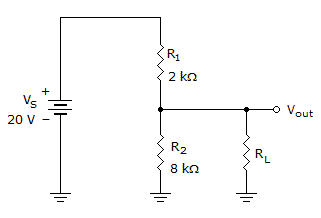# Electronics - Series-Parallel Circuits - Discussion

### Discussion :: Series-Parallel Circuits - General Questions (Q.No.3)

3.If the load in the given circuit is 120 k, what is the loaded output voltage?

 [A]. 4.21 V [B]. 15.79 V [C]. 16 V [D]. 19.67 V

Explanation:

No answer description available for this question.

 Yuvaraj said: (Feb 25, 2011) I need explanation for this problem.

 Nagamma said: (Mar 15, 2011) Which formula used to solve the problem?

 Priti said: (Mar 17, 2011) Please explain. Thank you.

 Ishwar said: (Mar 27, 2011) Voltage division principle is used. This way First determine the parallel combination of R2 and RL and then find the voltage division between the resultant and R1.

 Madhusudan said: (Jul 23, 2011) RL||R2=7.5k and vltg drop across 7.5k s (7.5/(7.5+2))*20=15.79...

 Karthik said: (Aug 20, 2011) In the Given Circuit RL=120K So RL and R2 in parallel, R2=8K 1/R=1/R2+1/RL 1/R=1/8+1/120 1/R=0.13333 R=7.5K THEN R AND R1 IN SERIES SO TOTAL R =9.5K CURRENT I =0.0021A SO VL = I*R VL=0.0021*7.5K VL =15.75.. Thanks, Karthik

 Amit said: (Sep 21, 2011) RL is given as 120k So 1st calculate the total resistance by 120k||8K=7500 then 2k+7500=9500 N v=ir 20=i*9500 i=20/9500=2.1052exp-3 Now by current division rule current through RL will be say I2=i*8k/(8k+120k)=1.315exp-4 again v=ir So say voltage across RL be v2 v2=1.315exp-4*120k =15.79v same can be solved using voltage division rule

 Rajesh Madavi said: (Jul 18, 2012) 8*120/8+120 =7.5k v=20*7.5k/7.5k+2k =20*15/19 =300/19 =15.79v

 Rekha said: (Nov 10, 2012) Very simple procedure, Step 1:Find parallel combination of 8K & 120K = 7.5K Step 2:Now 2k &7.5k are in series so total resistance=9.5k Step 3:Find ct I=20v/9.5k= 2.105mA, Step 4:find drop across 2K is 2.105m*2K=4.2V Step 5:Finally Vl=20-4.2=15.8v.

 Manoj said: (Jul 11, 2016) Thanks @Rekha.

 Yoosuf said: (Oct 28, 2016) Thanks for explaining the solution.

 Aravind said: (May 30, 2017) 8k parallel 10k= (8*120)/(8+120)= 7.5k. Now, Voltage across 7.5k = Vol.across 120k (bz 8k parallel 120k) So vol across 7.5k =(20V * 7.5k)/2k + 7.5k, = 15.79V. (Vol.divider equation)

 Vaishnavi said: (Jul 13, 2017) Thanks for explaining it.

 Pragadee said: (Jul 27, 2017) Thanks for explaining this.

 Shik Shansha Vali said: (Sep 15, 2017) RL = 120 anyone can explain?

 Vc_Davv said: (Nov 19, 2017) Don't be so complex. Use thevenin theorem. So vth = 16 v and Rth = 1.6 kohm. Voltage across Rl (load 120kohm) by VDL = (16*120K/120k+1.6k)=15.7v.

 Thiru said: (Feb 16, 2018) Can we choose this method? Apply *voltage Divider Rule * V=((Total voltage *opposite Resistance) /Total Resistance) 20*8/8+2=16V. Whatever the Voltage across parallel is same as the other side That's why its 16v.

 Alex said: (Dec 14, 2018) Is this series, parallel, or an open circuit? Please tell me.

 Akhil Va said: (Feb 27, 2019) resistor 120k is parallel with 8k. So r=1/120+1/8 = 7.5k. vout=r2/(r1+r2) = 7.5/((2+7.5). = 15.78947368421053 V, = 15.79 V.